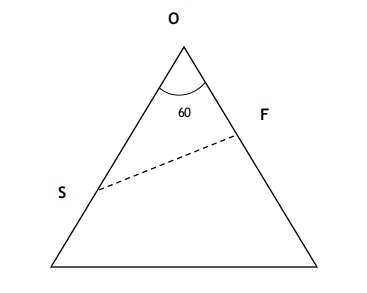Question 65

# Two ships are approaching a port along straight routes at constant speeds. Initially, the two ships and the port formed an equilateral triangle with sides of length 24 km. When the slower ship travelled 8 km, the triangle formed by the new positions of the two ships and the port became right-angled. When the faster ship reaches the port, the distance, in km, between the other ship and the port will be

SolutionLet S be the slower ship and F be the faster ship.

It is given that when S travelled 8 km, the positions of ships with the port is forming a right triangle.

Since one of the angles is 60(since one vertex is still part of the equilateral triangle),

the other two vertexes will have angles of 30 and 90.

The distance between O and S = 24 - 8 = 16

In triangle OFS, $$\cos60^0\ =\ \frac{OF}{OS}$$

Thus, OF = 8.

Thus in the time, S covered 8 km, F will cover 24 - 8 = 16 km.

Thus, the ratio of their speeds is 2:1,

Thus, when F covers 24 km, S will cover 12 km.

The correct option is B.